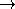## RectangularPCenterTraits_2

### Definition

The concept RectangularPCenterTraits_2 defines types and operations needed to compute rectilinear p-centers of a planar point set using the function rectangular_p_center_2.

### Types

 RectangularPCenterTraits_2::FT model for FieldNumberType. RectangularPCenterTraits_2::Point_2 model for Kernel::Point_2. RectangularPCenterTraits_2::Iso_rectangle_2 model for Kernel::Iso_rectangle_2. RectangularPCenterTraits_2::Less_x_2 model for Kernel::Less_x_2. RectangularPCenterTraits_2::Less_y_2 model for Kernel::Less_y_2. RectangularPCenterTraits_2::Construct_vertex_2 model for Kernel::Construct_vertex_2. RectangularPCenterTraits_2::Construct_iso_rectangle_2 model for Kernel::Construct_iso_rectangle_2. RectangularPCenterTraits_2::Signed_x_distance_2 adaptable binary function class: Point_2  × Point_2FT returns the signed distance of two points' x-coordinates. RectangularPCenterTraits_2::Signed_y_distance_2 adaptable binary function class: Point_2  × Point_2FT returns the signed distance of two points' y-coordinates. RectangularPCenterTraits_2::Infinity_distance_2 adaptable binary function class: Point_2  × Point_2FT returns the || · ||distance of two points. RectangularPCenterTraits_2::Signed_infinity_distance_2 adaptable binary function class: Point_2  × Point_2FT returns the signed || · ||distance of two points. RectangularPCenterTraits_2::Construct_point_2_below_left_implicit_point_2 3-argument function class: Point_2  × Point_2  × FTPoint_2. For arguments (p,q,r) it returns the lower-left corner of the iso-oriented square with sidelength r and upper-right corner at the intersection of the vertical line through p and the horizontal line through q. RectangularPCenterTraits_2::Construct_point_2_below_right_implicit_point_2 3-argument function class: Point_2  × Point_2  × FTPoint_2. For arguments (p,q,r) it returns the lower-right corner of the iso-oriented square with sidelength r and upper-left corner at the intersection of the vertical line through p and the horizontal line through q. RectangularPCenterTraits_2::Construct_point_2_above_right_implicit_point_2 3-argument function class: Point_2  × Point_2  × FTPoint_2. For arguments (p,q,r) it returns the upper-right corner of the iso-oriented square with sidelength r and lower-left corner at the intersection of the vertical line through p and the horizontal line through q. RectangularPCenterTraits_2::Construct_point_2_above_left_implicit_point_2 3-argument function class: Point_2  × Point_2  × FTPoint_2. For arguments (p,q,r) it returns the upper-left corner of the iso-oriented square with sidelength r and lower-right corner at the intersection of the vertical line through p and the horizontal line through q.

### Operations

For every function class listed above there is a member function to fetch the corresponding function object.

 Inf_distance_2 t.inf_distance_2_object () const Signed_inf_distance_2 t.signed_inf_distance_2_object () const Construct_vertex_2 t.construct_vertex_2_object () const Construct_iso_rectangle_2 t.construct_iso_rectangle_2_object () const Construct_iso_rectangle_2_below_left_point_2 t.construct_iso_rectangle_2_below_left_point_2_object () const Construct_iso_rectangle_2_above_left_point_2 t.construct_iso_rectangle_2_above_left_point_2_object () const Construct_iso_rectangle_2_below_right_point_2 t.construct_iso_rectangle_2_below_right_point_2_object () const Construct_iso_rectangle_2_above_right_point_2 t.construct_iso_rectangle_2_above_right_point_2_object () const

### Has Models

CGAL::Rectangular_p_center_default_traits_2<K>